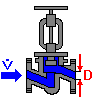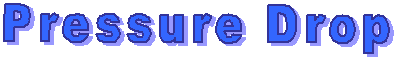## FAQ (frequently asked questions) on SF Pressure Drop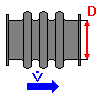### Calculations of Pressure Drops of gases - Comments

The pressure drop of gases is calculated with an approximate equation:

If you deliver gases the pressure drop causes an expansion of the gas, so the density will be lower and the velocity increases. Because of that the pressure drop isn't constant along the pipe.

If you have select the condition "gaseous" the program calculates the pressure drop with an approximate equation (pressure drop at arbitrary heat transfer). The program sets the pipe friction number as a constant and calculates it with the input data. The temperature, which is used in the equation, is the average of entrance and exit of pipe.

The program needs, to use this formula, for each element the incoming pressure, the incoming temperature and the leaving temperature. Please note, that the incoming pressure is smaller for the next elements. The program doesn't calculate a new incoming pressure, it uses the data in the window "Additional data for gases" which you have input. If you have select number of elements > 1 errors are possible, if the pressure drops are hight. In such cases it's necessary to calculate each element with its own inlet pressure, inlet temperature and outlet temperature.
The software can proceed this automatically. To activate this function use menu Extras/Settings part "Pressure drop calculations of gases/Refresh inlet pressure and gas properties" the choice "refresh with data from database (real gas)". If the gas is not in the database you can use the choice "refresh by calculation (ideal gas)". With this calculation we can get a good approximation normally, but it's not possible to update the viscosity of the gas.

You can calculate pressure drops of gases with the same formula as liquids if the relativ change of density is low (change of density/density = 0.02). Have you such a case then it's possible to calculate the gases as liquids. Switch in the "Flow medium" window the condition button to liquid and calculate in the normal way. If you do so you have the advantage that you don't need data of gas (pressure inlet, temperature inlet and outlet.### If I order the program as CD, need I the password additional?

If you order the program as CD, we deliver the needed password with the disk. The price for the disk contains the price for the password.
If you have the actual shareware version, you need to register the program the password only. You don't need a disk in this case.

Please note that the we can deliver the password by e-mail only.### I want calculate gases. Have I input the volume flow as normal or working volume flow?

The program needs to calculate pressure drops of gases the working volume flow. If you want input the flow as normal flow select the volume flow units Nm³/h, Nm³/min oder Nm³/s. The program converts this data in working-m³ with the formula for ideal gases. To convert the pressure and temperatur is needed. The program uses the values from the window "Additional data for gases". Low differences to the reality are possible.
Phase changes gas/liquid will not detect from the program.### I want calculate fittings. Can I use the kv or Cv value?

The kv value is the flow coefficient for valves, expresses flow rate in m3/h of water with 1 bar pressure drop across valve. In the USA the Cv value is used, expresses flow rate in gal/min of water with 1 lb/sq in (1 kv = 0.86 Cv; 1 Cv = 1.17 kv).

Resistance coefficient = (0.05 A / kv)² A in mm²

The kv or Cv value is valid for water only. You can convert it for other liquids in this way:

kv = flow rate x (density/pressure drop)^-2### Pressure Drop in valves (influence of viscosity; calculation as equivalent pipe-lenght)

In a valve are 2 pressure drops:

1.pressure drop caused by friction of the fluid at the wall of the valve.

2.ressure drop caused by turbulences and flow separation.

to 1:

The pressure drop caused by friction at the wall is small in the most cases and so we can disregard this pressure drop. In extrem conditions, many fittings, we have to consider this factor. We calculate the length of the fittings as a normal pipe.

zu 2:

The most part of pressure drop in valves is caused by turbulences and flow separation.

This pressure drop is a little only dependent on Reynolds number and viscosity so we can use the formula dp = resistance coefficient x velocity²/ 2 x density.

Experimental values you will find in "Resistance coefficients for laminar and turbulent flow through one-half-inch valves and fittings" in Transaction of the ASME (79, 1759 - 1766; 1957).

From this it can be concluded that you can calculate pressure drops in the whole turbulent area with the resistance coefficients. The pressure drop is no function from the viscosity.

The calcualation of pressure drops as equivalent pipe-lenght is correct only if the pressure drops in valves are in the main pressure drops caused by friction.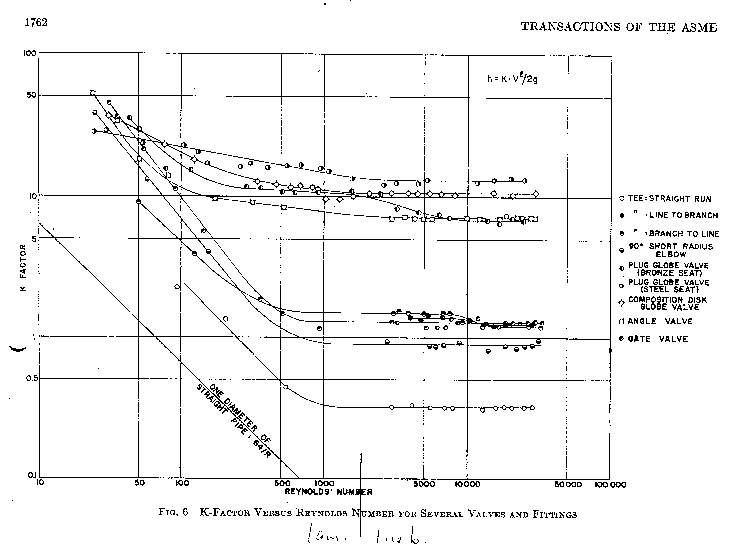### Can we use the program in our network?

The program can used in a network without problems. The settings of each user are saved separately. It's not possible that several users edit a project file at the same time.

It's not allowed to use the software by several users at the same time with an one user license. You can increase the number of users by ordering of further software packages.

When installed on a network, the number of concurrent users is limited to the number of licenses purchased.### Does the software calculate dynamic pressure changes of enlargements and contractions?

In enlarging or contracting pipes the following pressure changes are existing:

1. Pressure drop caused by friction, turbulences and flow separation:
dP = resistance coefficient x density/2 x velocity²

2. Pressure change caused by changes of kinetic energy acc. Bernoulli formula:
dP = density * (velocity1² - velocity2²)/2.
For enlargements the pressure change is positiv, for contractions negativ.

The element "Dyn. pressure change" calculates the static pressure changes caused by changes of kinetic energy. Normally you input the dimension of begin and end of the whole pipe.

Attention: The calculated data are output as pressure drop, i.e. if the output is negativ the pressure is encreasing, if the output is positiv the pressure is decreasing.

In previous program versions (< version 6.25) these pressure changes were calculated in combination with pipe enlargements and contractions.

Correlation: Static pressure, dynamic pressure and total pressure
and calculation example

(Pressure changes caused by friction, turbulences and vertical difference of pipe are not considered.)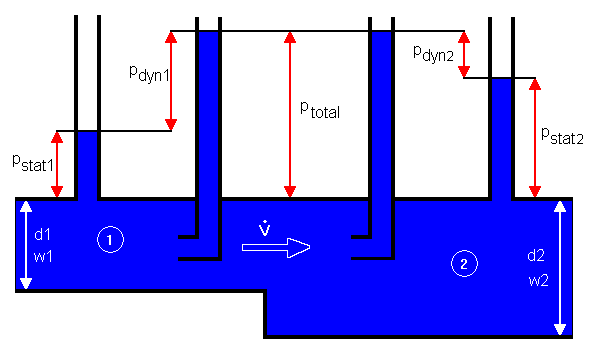In flows the following pressures are existing:

• static or hydrostatic pressure p_stat
• dynamic or velocity pressure p_dyn

The static pressure is static relative to the moving fluid and can be measured through a flat opening in parallel to the flow (pressure gauge).

The dynamic pressure is caused by kinetic energy, measurement method pitot-static tube.

The summary of static and dynamic pressure is the total pressure. It is measured by a Pitot tube.

According the formula of Bernoulli the sum of static and dynamic pressure is constant:

p_total1 = p_total2 = constant

p_stat1 + p_dyn1 = p_stat2 + p_dyn2 = constant

Die kinetic energy of the flow is called dynamic or velocity pressure. The following formula is valid:

p_dyn = density/2 x velocity²

Calculation example:

 Position 1 2 Diameter d mm 50 70 Volume flow V m³/h 25 Density kg/m³ 1000 Velocity w m/s 3.54 1.80 Dynamic pressure p_dyn bar 0.063 0.016 Static pressure p_stat bar 8 8.046 Total pressure p_total bar 8.063 p_stat2 - p_stat1 bar 0.046 Pressure drop (stat.) bar -0.046### Questions to the pipe element "Vertical difference"

To calculate the whole pressure drop for each element it would be necessary to detect the vertical difference between inlet and outlet. Because it would be a big effort we have input a own element "Vertical difference".

Please calculate vertical differences in the following way: Calculate all elements without consideration of the vertical difference. Finally insert the element "Vertical difference" with vertical distance of inlet and outlet of the whole pipe.### Can I open project files from previous program versions?

It's possible to open old project files but it's not possible to open new project files with an older program version.### Can I work in 2 project files at the same time?

Start the program SF Pressure Drop several times.### Calculates the program pressure drops caused by friction within bends?

The program takes the pressure drop caused by friction into account, hence it's necessary to input the pipe rougness. Other pressure drops are caused by changes of direction.### What I have to consider at the calculation of composed resistances (example bend + valve + strainer)?

If pipe elements are series-connected, which are calculated with resistance coefficients, so it's possible that because of the addition of the resistance coefficients errors are generated, because the inlet and outlet conditions are unequal the test conditions, in which the resistance coefficients were measured. Normally it would be necessary to measure each combination.### Notes for using the unit Nm3 of volume flow for gases

The program needs to calculate pressure drops of gases the working volume flow. If you want input the flow as normal flow select the volume flow units Nm3/h, Nm3/min oder Nm3/s. The program converts this data in working-m3 with the formula for ideal gases. To convert the pressure and temperatur is needed. The program uses the values from the window "Additional data for gases". Low differences to the reality are possible.
Phase changes gas/liquid will not detect from the program.### Important note to the windows "Additional data for gases" related properties of gases

Important: If you change the temperature or pressure of the gas in the window "Additional data for gases", the program doesn't actualise the density and viscosity in the window "Flow medium"! The user has input the new density and viscosity in this window (Menu Flow medium/properties).### How calulates the software pipes with non-circular diameter (hydraulic diameter)?

You can calculate pressure drops in pipes with non-circular diameter with the normal formula if you use instead the diameter the hydraulic diameter:

Hydraulic diameter = 4 * cross section area / circumference

Velocity = volume flow / cross section area

Reynolds number = velocity * hydraulic diameter * density / dyn. viscosity

Pipe friction number Lamda at turbulent flow: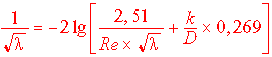Pressure drop = Lamda * pipe length / hydr. diameter * density / 2 * velocity²

As example a calculation of a rectangular pipe:

Known:

Width = 100 mm; Height = 50 mm; Length = 1 m

Pipe roughness k = 0.1 mm

Volume flow = 100 m³/h

Density = 998.2 kg/m³; Dyn. Viscosity = 1002 * 10^-6 kg/ms

Calculation:

Cross section area = 100 mm * 50 mm = 5000 mm²

Circumference = 2 * (100 mm + 50 mm) = 300 mm

Hydraulic diameter = 4 * 5000 mm² / 300 mm = 66.67 mm

Velocity = 100 m³/h / 5000 mm² = 5.56 m/s

Reynols number = 5.56 m/s * 66.67 mm * 998.2 kg/m³ / 1002 * 10^-6 kg/ms = 3.69 * 10^5

Pipe friction number = 0.022

Pressure drop = 0.022 * 1 m / 66.67 mm * 998.2 kg/m³ / 2 * (5.56 m/s)² = 51.544 mbar### Enlargements/contractions: If I change the opening agle, what's the result for pressure drop?

It's different whether we have laminar or turbulent flow.

Turbulent flow
The pressure drop in enlargements/contractions at turbulent flow is caused by turbulences within flow medium primarily. The pressure drop caused by friction at the wall of the pipe is marginal. If we increase the opening angle we have more turbulences and the pressure drop will increase.

Laminar flow
The pressure drop in enlargements/contractions at laminar flow is caused by friction at the wall of the pipe primarily. The pressure drop caused by turbulences within the flow medium is marginal. If we increase the opening angle the length of the pipe element will be smaller and the pressure drop will decrease.### Calculation of 90 degree angles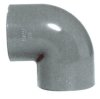I want calculate 90 degree angles, but the program shows 90 degree bends only (look image). What can I do?Please use the element "miter bend" in the group "bends". As angle input 90 degree and calculate.### What’s the meaning of „appr.“ at the boiling point in the window „Calculation of properties“?

In this case the boiling point calculation is out of the range of the used formula. To get a approximate value of the boiling point we use a rough estimation. The density and viscosity values in this range are to use conditionally.